## Abstract

A new method for model reduction of linear systems is presented, based on Chebyshev rational functions, using the Harmony Search (HS) algorithm. First, the full order system is expanded and then a set of parameters in a fixed structure are determined, whose values define the reduced order system. The values are obtained by minimizing the errors between the ${\textstyle l}$ first coefficients of the Chebyshev rational function expansion of full and reduced systems, using the HS algorithm. To assure stability, the Routh criterion is used as constraints in the optimization problem. To present the ability of the proposed method, three test systems are reduced. The results obtained are compared with other existing techniques. The results obtained show the accuracy and efficiency of the proposed method.

## Keywords

Chebyshev rational functions ; Harmony search algorithm ; Order reduction ; Orthogonal functions ; Routh array

## 1. Introduction

Reduced order models are highly desirable for engineers in system analysis, synthesis and simulation of complicated high-order systems, since the analysis and design of such systems is not an easy task. Various methods for model reduction are reported in the literature in the time and frequency domains. Model reduction was started by Davison in 1966   and followed by Chidambara, who suggested several modifications to Davison’s approach   ,   and  . After that, different approaches were proposed using dominant eigenvalue retention    and  , Routh approximation   , Hurwitz polynomial approximation    and  , the stability equation method    and  , moments matching  ,  ,   and  , the continued fraction method   ,   and  , Pade approximation   and etc.

The issue of optimality in model reduction was considered by Wilson    and  , who suggested an optimization approach based on minimization of the integral squared impulse response error between full and reduced-order models. This attempt was continued by other researchers through other approaches   ,  ,   and  .

In 1981   , the controllability and observability of the states were considered in model reduction by Moore. The suggested approach suffered from steady state errors but the stability of the reduced model was assured if the original system was also stable   . Furthermore, the concept of ${\textstyle H_{\infty }}$ , ${\textstyle H_{2}}$ , ${\textstyle L_{2}}$ and ${\textstyle L_{\infty }}$ were used for model reduction in   ,  ,   and  .

In recent decades, evolutionary techniques, such as Particle Swarm Optimization (PSO) and Genetic Algorithm (GA), have been used for order reduction of systems   ,   and  . In these approaches, the reduced order model parameters are achieved by minimizing a fitness function, which is often Integral Square Error (ISE), Integral Absolute Error (IAE), ${\textstyle H_{2}}$ norm or ${\textstyle H_{\infty }}$ norm   ,   and  .

This paper proposes an alternative method for order reduction based on Chebyshev rational functions, using the HS algorithm. The full order system is expanded and then the ${\textstyle l}$ first coefficients of Chebyshev rational function expansion are obtained. A desire fixed structure for the reduced order model is considered and a set of parameters are defined, whose values determine the reduced order system. These unknown parameters are determined using the harmony search algorithm by minimizing the errors between the ${\textstyle l}$ first coefficients of Chebyshev rational function expansion of full and reduced systems. To assure stability, the Routh criterion is applied, as used in   , where it states optimization problems as constraints, which, subsequently, are converted to constrained optimization problems. To show the accuracy of the proposed method, three systems are reduced by the proposed method and compared with those available in the literature.

To make a proper background, Chebyshev rational functions and the harmony search are briefly explained in Sections  2  and 3 , respectively. The proposed method is explained in Section  4 . The ability of the proposed approach is shown in Section  5 , and, finally, the paper is concluded in Section  6 .

## 2. The Chebyshev rational functions

The Chebyshev rational functions are a sequence of functions that are both orthogonal and rational   . A rational Chebyshev function of degree ${\textstyle l}$ is presented as:

 $R_{l}(x)=T_{l}({\frac {x-1}{x+1}}){\mbox{,}}$
( 1)

where ${\textstyle T_{l}(x)}$ is a Chebyshev polynomial and defined by a recursive formula as:

 $T_{0}(x)=1{\mbox{,}}$
( 2)

Using the orthogonality relationship, an arbitrary function, ${\textstyle f(x)}$ , can be expanded as:

 $f(x)=\sum _{l=0}^{\infty }F_{l}R_{l}(x){\mbox{,}}$
( 3)

where ${\textstyle F_{l}}$ is given by the following equations:

 $F_{l}={\frac {2}{c_{l}\pi }}\int _{0}^{\infty }f(x)R_{l}(x)W(x)dx{\mbox{,}}$
( 4)

in which, ${\textstyle c_{l}}$ equals 2 for ${\textstyle l=0}$ and ${\textstyle c_{l}}$ equals 1 for ${\textstyle l\geq 1}$ . Also, W is given as:

 $W(x)={\frac {1}{(x+1){\sqrt {x}}}}{\mbox{.}}$
( 5)

Therefore, by considering the first ${\textstyle l}$ terms of Eq. (3) , a good approximant of ${\textstyle f(x)}$ is obtained.

## 3. Harmony search algorithm

The HS is based on a natural musical process, which searches for a perfect state of harmony. In general, the HS algorithm works as follows    and  :

Step 1. Initialization: Define the objective function and decision variables, and input the system parameters and the boundaries of the decision variables. The optimization problem can be defined as:

Minimize ${\textstyle f(x)}$ subject to ${\textstyle x_{iL} where ${\textstyle x_{iL}}$ and ${\textstyle x_{iU}}$ are the lower and upper bounds for decision variables.

The HS algorithm parameters are also specified in this step. They are the Harmony Memory Size (HMS ) or the number of solution vectors in harmony memory, Harmony Memory Considering Rate (HMCR ), distance bandwidth (bw ), Pitch Adjusting Rate (PAR ), and the number of improvisations (K ), or stopping criterion. K is the same as the total number of function evaluations.

Step   2. Initialize the harmony memory (HM). The harmony memory is a memory location where all the solution vectors (sets of decision variables) are stored. The initial harmony memory is randomly generated in the region ${\textstyle [x_{iL},x_{iU}](i=1,2,\ldots ,N)}$ . This is done based on the following equation:

 $x_{i}^{j}=x_{iL}+rand()\times (x_{iU}-x_{iL})\quad j=1,2,\ldots ,HMS{\mbox{,}}$
( 6)

where ${\textstyle rand()}$ is a random from a uniform distribution of ${\textstyle [0,1]}$ .

Step   3. Improvise a new harmony from the harmony memory. Generating a new harmony, ${\textstyle x_{i}^{new}}$ , is called improvisation, which is based on three rules: memory consideration, pitch adjustment and random selection. First of all, a uniform random number, r   , is generated in the range ${\textstyle [0,1]}$ . If r   is less than HMCR, the decision variable, ${\textstyle x_{i}^{new}}$ , is generated by the memory consideration; otherwise, ${\textstyle x_{i}^{new}}$ is obtained by a random selection. Then, each decision variable, ${\textstyle x_{i}^{new}}$ , will undergo a pitch adjustment with a probability of PAR if it is produced by the memory consideration. The pitch adjustment rule is given as follows:

 $x_{i}^{new}=x_{i}^{new}\pm r\times bw{\mbox{.}}$
( 7)

Step   4. Update harmony memory. After generating a new harmony vector, ${\textstyle x^{new}}$ , the harmony memory will be updated. If the fitness of the improvised harmony vector, ${\textstyle x^{new}=(x_{1}^{new},x_{2}^{new},\ldots ,x_{N}^{new})}$ , is better than that of the worst harmony, the worst harmony in the HM will be replaced with ${\textstyle x^{new}}$ and become a new member of the HM.

Step 5. Repeat Steps 3–4 until the stopping criterion (maximum number of improvisations K ) is met.

## 4. The proposed model reduction method

Consider a stable Single-Input Single-Output (SISO) system described by the transfer function of order n as follows:

 $G(s)={\frac {a_{1}s^{n-1}+a_{2}s^{n-2}+\cdots +a_{n}}{s^{n}+b_{1}s^{n-1}+b_{2}s^{n-2}+\cdots +b_{n}}}{\mbox{,}}$
( 8)

where ${\textstyle a_{i}}$ and ${\textstyle b_{i}}$ are constants.

The objective is to obtain a reduced model of order r , where r is smaller than n , such that the principal and important specifications of the full order system are retained in the reduced order model. This reduced order system is presented as follows:

 $G_{r}(s)={\frac {c_{1}s^{r-1}+c_{2}s^{r-2}+\cdots +c_{r}}{s^{r}+d_{1}s^{r-1}+d_{2}s^{r-2}+\cdots +d_{r}}}{\mbox{,}}$
( 9)

where ${\textstyle c_{1},c_{2},\ldots ,c_{r}}$ and ${\textstyle d_{1},d_{2},\ldots ,d_{r}}$ are unknown constants.

To obtain the reduced model by the proposed method, firstly, the full order system is expanded based on Chebyshev rational functions. Then, the ${\textstyle l}$ first coefficients of the Chebyshev rational function expansion of the original system are obtained and shown by ${\textstyle F_{i}i=0,1,2,3,\ldots ,l}$ . Then, a desired fixed structure is considered for the reduced order model, as defined in Eq. (9) , where ${\textstyle c_{1},c_{2},\ldots ,c_{r}}$ and ${\textstyle d_{1},d_{2},\ldots ,d_{r}}$ are unknown parameters of the reduced order model that are obtained by HS. The goal of the optimization is to find the best parameters for ${\textstyle G_{r}(s)}$ . Therefore, each harmony is a ${\textstyle d}$ -dimensional vector, in which ${\textstyle d}$ is ${\textstyle c_{r}+d_{r}}$ . Each harmony is a solution to ${\textstyle G_{r}}$ and for each solution (harmony), the Chebyshev rational function expansions are obtained. Each harmony is evaluated by minimizing the following fitness function:

 $J^{_{\ast }}=\sum _{i=0}^{l}(\vert F_{i}-{\overset {\mbox{ˆ}}{F}}_{i}\vert ){\mbox{,}}$
( 10)

in which ${\textstyle {\overset {\mbox{ˆ}}{F}}_{i}}$ are the coefficients of the Chebyshev rational function expansions of the reduced order system. The algorithm searches for the best harmony until the termination criteria are met. At this stage, the best parameters are given as parameters of the reduced order model.

Furthermore, the reduced model must be stable if the original system is stable. Therefore, the Routh criterion is applied to assure stability. For specifying the stability conditions, following   , the denominator polynomial of the reduced order model in Eq. (9) can be shown as below:

 ${\begin{array}{l}\displaystyle s^{r}+h_{1}s^{r-1}+(h_{2}+h_{3}+\cdots +h_{r})s^{r-2}+h_{1}(h_{3}+h_{4}+\cdots +\\\displaystyle +h_{r})s^{r-3}+[h_{2}(h_{4}+h_{5}+\cdots +h_{r})+h_{3}(h_{5}+h_{6}+\cdots +h_{r})+\\\displaystyle +h_{4}(h_{6}+h_{7}+\cdots +h_{r})+\cdots h_{r-2}h_{r}]s^{r-4}+\cdots +\\\displaystyle +h_{1+q}h_{3+q}\cdots h_{r-2}h_{r}{\mbox{,}}\end{array}}$
( 11)

which is constructed by taking the coefficients of the first two rows of the Routh array, in which elements of its first column have the following entries:

 $1,h_{1},h_{2},h_{1}h_{3},h_{2}h_{4},h_{1}h_{3}h_{5},\ldots ,h_{1+k}h_{3+k}...h_{r-2}h_{r}{\mbox{,}}$
( 12)

where k is equal to 1 for even r , and k is equal to 0 for odd r .

Comparing the entries of the array in Eq. (12) with ${\textstyle 1,d_{2},d_{4},\ldots }$ and those of the second row with ${\textstyle d_{1},d_{3},d_{5},\ldots }$ gives Eq. (13) :

 ${\begin{array}{c}d_{1}=h_{1}\\d_{2}=(h_{2}+h_{3}+\cdots +h_{r})\\d_{3}=h_{1}(h_{3}+h_{4}+\cdots +h_{r})\\\vdots \\d_{r}=(h_{1+k}h_{3+k}h_{r-2}h_{r}){\mbox{.}}\end{array}}$
( 13)

Substituting the above relations in the reduced order model denominators, Eq. (11) is achieved. Therefore, the necessary and sufficient condition for all roots of the reduced system to be strictly in the left-half plane is:

 ${\begin{array}{c}h_{1}>0\\h_{2}>0\\\vdots \\h_{r}>0{\mbox{,}}\end{array}}$
( 14)

and, subsequently:

 ${\begin{array}{c}d_{1}>0\\d_{2}>0\\\vdots \\d_{r}>0{\mbox{.}}\end{array}}$
( 15)

Thus, to have an optimum stable reduced system, the reduced order model’s parameters are determined by minimizing the following fitness function:

 ${\begin{array}{c}J=\sum _{i=0}^{l}(\vert F_{i}-{\overset {\mbox{ˆ}}{F}}_{i}\vert )\\{\mbox{subject to }}d_{j}>0{\mbox{ for }}j=1,\ldots ,r{\mbox{.}}\end{array}}$
( 16)

Therefore, the reduced order model is achieved, such that the ${\textstyle l}$ first coefficients of the Chebyshev rational function expansions of the full order system are equal to the ${\textstyle l}$ first coefficients of the Chebyshev rational function expansions of the reduced order model.

The proposed method can be summarized in the following steps:

Step 1: The Chebyshev rational functions of the full order system in Eq. (8) are obtained.

Step 2: A desire fixed structure is considered for the reduced order model, as defined in Eq. (9) , where ${\textstyle c_{1},c_{2}\ldots ,c_{r}}$ and ${\textstyle d_{1},d_{2},\ldots ,d_{r}}$ are unknown parameters of the reduced order model that are obtained in the next step.

Step 3: To obtain the unknown parameters, HS is applied. The goal of the optimization is to find the best parameters for ${\textstyle G_{r}(s)}$ . Therefore, each harmony is a ${\textstyle d}$ -dimensional vector, in which ${\textstyle d}$ is ${\textstyle c_{r}+d_{r}}$ . Each harmony is a solution to ${\textstyle G_{r}}$ and, for each solution (harmony), the Chebyshev rational functions are obtained. Each harmony is evaluated using the objective function defined by Eq. (16) , searching for the harmony associated with ${\textstyle J_{best}}$ until the termination criteria are met. At this stage, the best parameters are given as parameters of the reduced order model.

## 5. Simulation and results

To assess the efficiency of the proposed approach, it has been applied to three test systems, where a step-by-step procedure is given for the first test system.

Test system 1: The first system to be reduced is a system of order 6 given by Mukherjee in   , where he uses a response matching technique to obtain the reduced system. ${\textstyle G(s)}$ is given in Box I .

 ${\begin{array}{l}\displaystyle G(s)=\\\displaystyle ={\frac {s^{5}+1014s^{4}+14069s^{3}+69140s^{2}+140100s+100000}{s^{6}+222s^{5}+14541s^{4}+248420s^{3}+1454100s^{2}+2220000s+1000000}}{\mbox{.}}\end{array}}$
( 17)

The reduced-order model can be obtained by the following steps:

Step 1: Based on Section  2 , the Chebyshev rational function of the full order system in Eq. (17) is obtained as:

 ${\begin{array}{l}\displaystyle G(s)=(0.0578)(1)+(-0.0256)({\frac {s-1}{s+1}})+\\\displaystyle +(-0.0195)({\frac {s^{2}-6s+1}{(s+1)^{2}}})+(0.0011)({\frac {s^{3}-15s^{2}+15s-1}{(s+1)^{3}}})+\\\displaystyle +(-0.0198)({\frac {s^{4}-28s^{3}+70s^{2}-28s+1}{(s+1)^{4}}})\end{array}}$
( 18)

where the fifth order (${\textstyle l=5}$ ) Chebyshev rational function of Eq. (17) is considered to present ${\textstyle G(s)}$ .

Step 2: The full order of the system represented in Eq. (17) is going to be reduced to a third-order system with the following transfer function:

 $G_{r}(s)={\frac {c_{0}s^{2}+c_{1}s+c_{2}}{s^{3}+d_{0}s^{2}+d_{1}s+d_{2}}}{\mbox{,}}$
( 19)

where ${\textstyle c_{0},c_{1},c_{2}}$ and ${\textstyle d_{0},d_{1},d_{2}}$ are unknown parameters of the reduced order model.

Step 3: HS is applied to obtain the unknown parameters in Eq. (19) . Since, the goal of the optimization is to find the best parameters for ${\textstyle G_{r}(s)}$ , each harmony is a ${\textstyle d}$ -dimensional vector in which ${\textstyle d=6}$ . The HMS is selected to be 6, and the HMCR   and evaluation number are set to be 0.9 and 1000, respectively. Each harmony is a solution to ${\textstyle G_{r}}$ and, for each solution (harmony), the Chebyshev rational functions are obtained. Each harmony is evaluated using the objective function defined by Eq. (16) , searching for the best ${\textstyle J}$ , until the termination criteria are met. At this stage, the best parameters are given for the reduced order model, where the following reduced order model is obtained:

 $G_{r}(s)={\frac {4.55s^{2}+27.2s+50.78}{s^{3}+54.86s^{2}+691.56s+507.67}}{\mbox{.}}$
( 20)

The Chebyshev rational functions of the obtained reduced order model are as:

 ${\begin{array}{l}\displaystyle G_{r}(s)=(0.0578)(1)+(-0.0256)({\frac {s-1}{s+1}})+\\\displaystyle +(-0.0195)({\frac {s^{2}-6s+1}{(s+1)^{2}}})+(0.0011)({\frac {s^{3}-15s^{2}+15s-1}{(s+1)^{3}}})+\\\displaystyle +(-0.0198)({\frac {s^{4}-28s^{3}+70s^{2}-28s+1}{(s+1)^{4}}}){\mbox{.}}\end{array}}$
( 21)

Comparing Eqs. (18)  and (21) shows that the best approximant of ${\textstyle G(s)}$ is achieved. The step response of the full order system and that of the system with a third-order reduced model is shown in Figure 1 . This figure shows that the obtained reduced order model is an adequate low-order model that retains the characteristics of a full order model. Also, to show the efficiency of the proposed method, the step and frequency responses of the obtained reduced model are compared with those available in the literature. Figure 1  and Figure 2 show a comparison of the results obtained with the proposed method by Mukherjee   , Optimal Hankel norm approximation (HSV)   and Balanced Truncation (BT)   , respectively. It should be noted that a reduced order model is called a balanced truncation of the full order system, when a system is balanced and, then, the states corresponding to small Hankel singular values are discarded. Also, Optimal Hankel norm approximation finds a reduced order model, ${\textstyle G_{r}}$ (of degree r   ), such that the Hankel norm of the approximation error ${\textstyle (\Vert G-G_{r}\Vert _{H})}$ is minimized.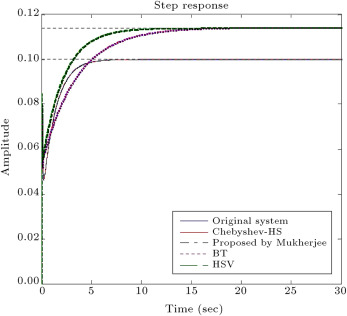Figure 1. The step response of full order and reduced order model by the proposed method and other methods for test system 1.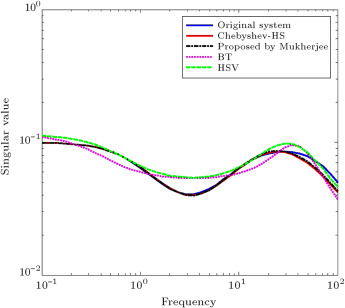Figure 2. The fequency response of full order and reduced order model by the proposed method and other methods for test system 1.

Figure 1  and Figure 2 show that the achieved results from the proposed method and the proposed method by Mukherjee are very similar to the original system, with respect to HSV and BT methods.

Furthermore, the specifications of the obtained system by the proposed method, such as steady state value, rise time, settling time and maximum overshoot, are compared with those obtained by Mukherjee   , HSV and BT, shown in Table 1 . Also, Integral Square Error (ISE) and the ${\textstyle H_{\infty }}$ norm of the error between the step responses of full order and reduced order models (${\textstyle e=\vert y-y_{r}\vert }$ ) are given in Table 1 . It is clearly seen that the specifications of the reduced order model that is achieved by the proposed method and the one by Mukherjee are close to the specifications of the original system.

Table 1. Comparision of methods for test system 1.
Model Steady state value Over shoot (%) Rise time (s) Settling time (s) ISE Infinity-norm of error
Full order 0.1 0 2.44 4.34
Chebyshev-HS 0.1 0 2.45 4.51 1.59×10−6 0.0067
Mukherjee 0.1 0 2.45 4.5 1.34×10−6 0.0074
BT 0.114 0 5.83 11.4 0.0379 0.0141
HSV 0.114 0 3.63 7.07 0.0375 0.0139

Also, the plot of ${\textstyle e=\vert y-y_{r}\vert }$ is given for the full order and reduced systems in Figure 3 . This figure illustrates that the obtained error by the proposed method in this paper and the proposed method by Mukherjee is very similar, and less than HSV and BT methods.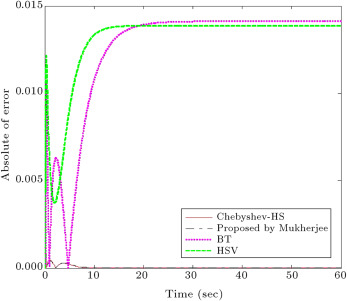Figure 3. The plot of ${\textstyle e=\vert y-y_{r}\vert }$ for the full order and reduced systems by the proposed method and other methods for test system 1.

Test system 2: In   , a procedure is presented to obtain a reduced order system by Routh–Pade approximation using the Luus–Jaakola algorithm. To compare the proposed method in this paper with the Luus–Jaakola algorithm, the system given in   is adopted, which is a third-order system:

 $G_{org}={\frac {8s^{2}+6s+2}{s^{3}+4s^{2}+5s+2}}{\mbox{.}}$
( 22)

Based on the explanations given for test system 1, the obtained reduced system by the algorithm is as follows:

 $G_{Chebyshev{\mbox{-}}HS}={\frac {7.88s+4.94}{s^{2}+3.64s+4.99}}{\mbox{.}}$
( 23)

The step response of the original system and the obtained reduced model is shown in Figure 4 . In this figure, the responses of the system with second-order primary reduced models obtained by other methods are also included for comparison. Also, the plot of ${\textstyle e=\vert y-y_{r}\vert }$ is given for the full order and reduced systems in Figure 5 .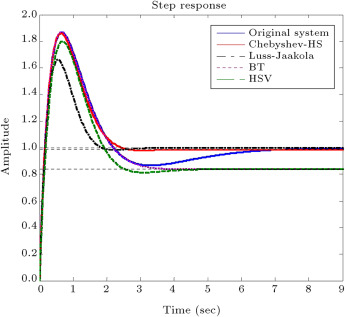Figure 4. The step response of full order and reduced order model by the proposed method and other methods for test system 2.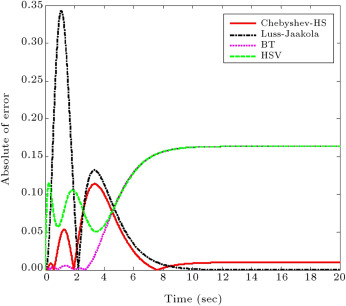Figure 5. The plot of ${\textstyle e=\vert y-y_{r}\vert }$ for the full order and reduced systems by the proposed method and other methods for test system 2.

Once again, maximum overshoot, rise time, settling time, steady state value, ISE and ${\textstyle H_{\infty }}$ norm of the error (${\textstyle e=\vert y-y_{r}\vert }$ ) are given in Table 2 . It is clearly seen that the specifications of the reduced order model achieved by the proposed method are close to the specifications of the original system, and better than other methods.

Table 2. Comparision of methods for test system 2.
Steady state value Overshoot (%) Rise time (s) Settling time (s) ISE Infinity-norm of error
Original model 1 86.5 0.129 6.74
Chebyshev- HS 0.99 87.9 0.127 2.35 0.0286 0.1139
Luus–Jaakola 1 66.1 0.13 1.71 0.1404 0.3425
BT 0.836 123 0.103 3.15 0.3802 0.1635
HSV 0.836 115 0.118 3.44 0.4043 0.1635

Test system 3: The third system to be reduced is a system given in   by Tao, where a procedure is presented to obtain a reduced system. ${\textstyle G_{Original}}$ is given in Box II .

 ${\begin{array}{l}\displaystyle G_{Original}=\\\displaystyle ={\frac {0.686(s+53)(s-53)(s^{2}-152.2s+14500)(s^{2}+153.8s+14500)}{(s^{2}+s+605)(s^{2}+45.5s+2660)(s^{2}+2.51s+3900)(s^{2}+3.99s+22980)}}{\mbox{.}}\end{array}}$
( 24)

Based on the explanations given for test system 1, the obtained reduced system by the algorithm is the one which is shown in Box III .

 ${\begin{array}{l}\displaystyle G_{Chebyshev}=\\\displaystyle ={\frac {0.012s^{4}-0.11s^{3}+230.02s^{2}+600.15s-303893.23}{s^{5}+59.88s^{4}+4142.24s^{3}+218504.11s^{2}+2246670.14s+1.08}}{\mbox{.}}\end{array}}$
( 25)

The comparison of the proposed method, the one proposed by Tao in   , HSV and BT methods are shown in Figure 6  and Figure 7 and Table 3 , which illustrate that the achieved results from the proposed method and the proposed method by Tao are very similar to the original system, with respect to HSV and BT methods.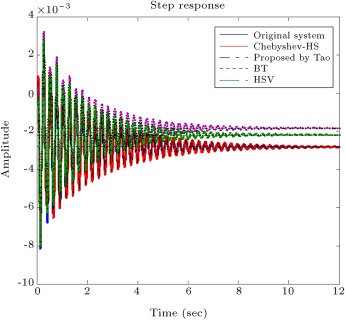Figure 6. Step response of full order and reduced order model by the proposed method and other methods for test system 3.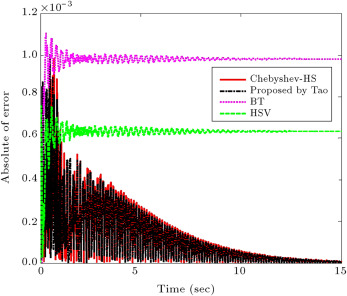Figure 7. The plot of ${\textstyle e=\vert y-y_{r}\vert }$ for the full order and reduced systems by the proposed method and other methods for test system 3.

Table 3. Comparision of methods for test system 3.
Steady state value Over shoot (%) Rise time (s) Settling time (s) ISE Infinity-norm of error
Original system −0.00281 −0.00814 0.0185 7.56
Chebyshev-Hs −0.00281 −0.0078 0.0164 9.13 5.8043×10−7 9.8248×10−4
Tao method −0.00281 −0.00738 0.0236 9.13 5.8235×10−7 7.5718×10−4
BT −0.00183 −0.00743 0.0146 7.31 1.4339×10−5 0.0011
HSV −0.00218 −0.00805 0.0719 7.18 5.9557×10−6 0.0010

## 6. Conclusion

This paper introduces a new method for order reduction, using orthogonal polynomials, through Chebyshev rational functions and the harmony search algorithm. To get an optimum stable reduced system, Routh array is applied as constraints in the formulation. The proposed method is compared with some order reduction techniques, where the results obtained show that the proposed approach has high accuracy, and which results in an adequate low-order model that retains the characteristics of the full order model. The obtained results confirm that the proposed method can be used as an alternative method for order reduction, and the ability of other orthogonal functions can be investigated for the order reduction of the system.

### Document informationPublished on 06/10/16

Licence: Other

### Document Score0

Views 34
Recommendations 0